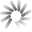\$11,109.97 +0.96 %
\$385.18 -0.38 %
\$93.96 -0.22 %
\$72.67 -0.53 %
\$58.82 -0.87 %
\$48.74 -0.03 %# Logicoin Hashrate Chart

The Logicoin hashrate chart provides the current Logicoin hashrate history in graph format with an option to expand the Logicoin global hashrate chart time frame back to 2014.

## Logicoin Hashrate Now: 6.68 KH/sJan 09, 2015 10:25 AM UTC - 6,679 H/s

Warning: Logicoin is no longer being monitored as of 1/9/2015.

##Loading Logicoin network hashrate chart

The Logicoin network hashrate chart can be used to visualize Logicoin mining hashrate increases and decreases viewable in segment options of daily, weekly, monthly, 3 months, 6 months, 1 year, 3 years, and all time.

## What is Logicoin Hashrate?

Logicoin hashrate is a calculated numerical value that specifies an estimate of how many hashes are being generated by Logicoin miners trying to solve the current Logicoin block or any given block.

Logicoin hashrate is represented in Hashes per Second or H/s.

The global Logicoin network hashrate is a calculated value and is measured in hashes per second (H/s). The calculation uses the current mining difficulty and the average Logicoin block time between mined blocks versus the defined block time as variables to determine the global Logicoin network hashrate.

As the Logicoin network hashrate goes up - the LGC hashrate numbers get so large that abbreviations must be used.

The abbreviations are SI derived units representing the number of hashes performed in a one second time frame.

### The current Logicoin hashrate is 6.68 KH/s, representing the global Logicoin network hashrate with a mining difficulty of 0.00 at block height 336,861.

Hashrate Unit/s Hash Hashes Per Second
H/s (Hash) 1 One
kH/s (KiloHash) 1,000 One Thousand
MH/s (MegaHash) 1,000,000 One Million
GH/s (GigaHash) 1,000,000,000 One Billion
TH/s (TeraHash) 1,000,000,000,000 One Trillion
EH/s (ExaHash) 1,000,000,000,000,000,000 One Quintillion
ZH/s (ZettaHash) 1,000,000,000,000,000,000,000 One Sextillion
YH/s (YottaHash) 1,000,000,000,000,000,000,000,000 One Septillion

It is important to point out the Logicoin hashrate does not determine how quickly or slowly each block is solved.

This timing, called the block time is enforced by the Logicoin mining difficulty value, which is adjusted upwards or downwards during each block difficulty retarget to keep blocks being solved at a constant time frame.

You can calculate Logicoin mining profits using the current LGC hashrate difficulty and our Logicoin hashrate calculator.

## What is the Current Logicoin Hashrate?

The current Logicoin hashrate (LGC hashrate) is 6.68 KH/s at block height 336,861 with a difficulty of 0.00.

Logicoin Hashrate Stats

### Current Logicoin Hashrate

Logicoin Global Hashrate
6.68 KH/s

### Logicoin Hashrate All Time High

Logicoin Hashrate on Sep 10, 2014 at block 203,513
1.16 GH/s

## How to Calculate Logicoin Hashrate

The Logicoin hashrate is calculated using the current Logicoin difficulty, the defined Logicoin block time, and the average block time of the last (X) number of blocks.

Most full Logicoin nodes will have an option to see the current Logicoin global hashrate using the "getnetworkhashps" command in the console window.Given the fact that the Logicoin difficulty value determines statically how many hashes are needed to solve the next block, it is recommended to use the current mining difficulty to determine the statistically accurate number of Logicoin that can be mined in a given time frame.

Once again we recommend using our Logicoin hashrate difficulty calculator as the current Logicoin difficulty is preloaded, along with the latest Logicoin price.

N/A
\$0.00 (0.00 %)

24 hour change